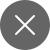# Data Science Interview Questions – Part 1

4+

Let’s begin with the questions.

Q.1    What is Data Science?

Data science is a comprehensive approach to extract useful insights from the massive and ever-increasing volumes of collected data. Data science involves data preparation for analysis and processing, performing advanced data analysis, and discussing the results to unveil patterns and facilitate stakeholders to form informed conclusions.

Q.2 Mention the differences between supervised and unsupervised learning?

A supervised machine learning algorithm aims to attain low bias and low variance. The algorithm will achieve good prediction performance then.

We can observe a general trend as:

• Linear machine learning algorithms usually have a high bias but low variance.
• Nonlinear machine learning algorithms usually have a low bias but high variance.

Below are two examples elaborating more on the bias-variance trade-off for specific algorithms:

• The k-nearest neighbors algorithm possesses low bias and high variance. Still, we can change the trade-off by increasing the value of K. It increases the number of neighbors contributing to the prediction and increases the model’s bias.
• The svm (support vector machine) possesses low bias and high variance. However, we can change the trade-off by tuning the C parameter that influences the training data’s margin, increasing bias, and decreasing the variance.

There is no avoiding the correlation between bias and variance in machine learning. This relationship is termed as bias-variance trade-off.

• On increasing the bias, the variance will decrease.
• Similarly, on increasing the variance, the bias will decrease.

Q.3 What is sampling? Explain different sampling methods?

Sampling is a technique that lets us get data about the population based on the statistics from a part of the population,i.e., sample. Sampling reduces our efforts in investigating every individual.

Sampling helps us to conclude given data using samples. It allows us to determine a population’s features by instantly seeing only a sample of the population.

## Different Types of Sampling Methods

We have two types of sampling methods:

• Probability sampling comprises random selection, enable you to make statistical inferences about the whole data.
• A non-probability sampling follows the approach of selecting non-random values based on need or other parameters. It enables you to collect starting data easily.

Q.4 What is linear regression? Also, discuss the assumptions of linear regression?

Linear regression is one of the simplest and very famous machine Learning algorithms. It is a statistical approach used for predictive analysis. Linear regression predicts continuous or numeric variables like prices, salary, age, sales, etc. Linear regression algorithm presents a linear relation between a  target (y) and one or more independent (y) variables.

Linear regression helps us to determine the contribution of input features for predicting the target variable. The linear regression model presents a sloped straight line. It shows the relationship between the variables. Have a look at the given image.

Linear regression can be mathematically represented as:

y = mx+c

Assumptions of Linear regression

The most important assumptions of linear regression are :

• The independent variables and the target variables should hold a linear relationship.
• There should be no multicollinearity among the features.
• Residuals(error terms) are normally distributed.
• Linear regression assumes no autocorrelation in residuals or error terms.

Q.5  How will you decide which machine learning algorithms will be a great fit for the given dataset?

It completely depends on the given dataset, which algorithm will work fine. We prefer linear regression for the. If the given data is non-linear, then the bagging algorithm will work fine. We can use decision trees or SVM if some interpretation is to be done regarding business purpose. Neural networks would be helpful if the dataset consists of images, audio, video, etc.

Hence, there is no reliable metric to determine which algorithm to be used for a given scenario or the data set. We need to understand the data using EDA (Exploratory Data Analysis). That’s why it is necessary to read all the algorithms in depth.

Q.6 Explain confusion matrix?

A confusion matrix is a table that evaluates the performance of a classification algorithm. It is a better method to evaluate the performance of your classification model.

That’s it for this article. We will be covering questions like this in our next article. So, make sure to stay connected with us.4+

### 2 Responses

1. January 31, 2021

[…] have covered some essential data science interview questions in our previous article. In this article also, I’ll be covering more such […]

0
2. March 10, 2021

[…] check Data Science Interview Questions – Part 1 and Data Science Interview Questions – Part […]

0